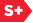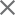### Proceedings Paper

Fast DCT-based image convolution algorithms and application to image resampling and hologram reconstruction
Format Member Price Non-Member Price
PDF \$17.00 \$21.00

Paper Abstract

Convolution and correlation are very basic image processing operations with numerous applications ranging from image restoration to target detection to image resampling and geometrical transformation. In real time applications, the crucial issue is the processing speed, which implies mandatory use of algorithms with the lowest possible computational complexity. Fast image convolution and correlation with large convolution kernels are traditionally carried out in the domain of Discrete Fourier Transform computed using Fast Fourier Transform algorithms. However standard DFT based convolution implements cyclic convolution rather than linear one and, because of this, suffers from heavy boundary effects. We introduce a fast DCT based convolution algorithm, which is virtually free of boundary effects of the cyclic convolution. We show that this algorithm have the same or even lower computational complexity as DFT-based algorithm and demonstrate its advantages in application examples of image arbitrary translation and scaling with perfect discrete sinc-interpolation and for image scaled reconstruction from holograms digitally recorded in near and far diffraction zones. In geometrical resampling the scaling by arbitrary factor is implemented using the DFT domain scaling algorithm and DCT-based convolution. In scaled hologram reconstruction in far diffraction zones the Fourier reconstruction method with simultaneous scaling is implemented using DCT-based convolution. In scaled hologram reconstruction in near diffraction zones the convolutional reconstruction algorithm is implemented by the DCT-based convolution.

Paper Details

Date Published: 4 May 2010
PDF: 7 pages
Proc. SPIE 7724, Real-Time Image and Video Processing 2010, 77240N (4 May 2010); doi: 10.1117/12.854669
Show Author Affiliations
Leonid Bilevich, Tel Aviv Univ. (Israel)
Leonid Yaroslavsky, Tel Aviv Univ. (Israel)

Published in SPIE Proceedings Vol. 7724:
Real-Time Image and Video Processing 2010
Nasser Kehtarnavaz; Matthias F. Carlsohn, Editor(s)